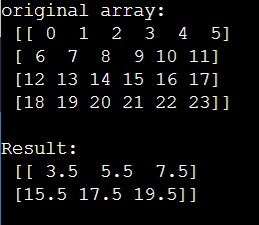# Resize with averaging or rebin a NumPy 2d array

Learn, how to resize with averaging or rebin a NumPy 2d array in Python? By Pranit Sharma Last updated : April 04, 2023

Suppose that we are given a 2D numpy array of shape 4X6 and we need to resize this array to 2X3 by taking the mean of some mean of the relevant samples.

## NumPy 2D Array - Resize with averaging or rebin

To resize with averaging or rebin a NumPy 2d array, we simply use numpy.reshape() method. It gives a new shape to an array without changing its data. The new shape should be compatible with the original shape. Inside this function we can pass the required dimensions and, we will apply the mean function on this with for a relevant row of this array.

Let us understand with the help of an example,

## Python code to resize with averaging or rebin a NumPy 2d array

```# Import numpy
import numpy as np

# Creating a numpy array
arr = np.arange(24).reshape((4,6))

# Display original array
print("original array:\n",arr,"\n")

# Reshaping the array
res = arr.reshape((2,arr.shape//2,3,-1)).mean(axis=3).mean(1)

# Display result
print("Result:\n",res)
```

### Output